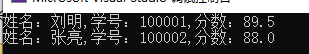m0_69827971 2022-09-29 08:54 采纳率: 60%

# 求各位大神带一带 真的不是很会

ShowInfo方法用于显示每个学生类对象的成员变量信息。

（提示：按类文件+实现文件+主文件格式书写代码）

• 写回答

#### 2条回答默认 最新

•qfl_sdu 2022-09-29 09:05
关注

题目给你说的比较清楚了，代码如下：
Student.h (类文件)

``````#pragma once

#include <iostream>
#include <cstring>
#include <iomanip>
using namespace std;
class Student
{
private:
char name;//姓名
char id;  //学号
float score;  //分数
public:
//无参构造函数
Student();

//3参数构造函数
Student(const char _name[], const char _id[], float s);

void ShowInfo();

};

``````

Student.cpp（实现文件）

``````#include "Student.h"

Student::Student()
{
name = 0;
id = 0;
score = 0;
}
//3参数构造函数
Student::Student(const char _name[], const char _id[], float s)
{
strcpy_s(name, 40,_name);
strcpy_s(id,20, _id);
score = s;
}
void Student::ShowInfo()
{
cout << "姓名：" << name << ",学号：" << id << ",分数：";
cout << fixed << setprecision(1) << score << endl; //保留1位小数
}

``````

main.cpp（主文件）

``````#include "Student.h"
using namespace std;

int main()
{
Student lm("刘明", "100001", 89.5);
Student zl("张亮", "100002", 88);
lm.ShowInfo();
zl.ShowInfo();
}

``````
本回答被题主选为最佳回答 , 对您是否有帮助呢?
评论 编辑记录

#### 悬赏问题

• ¥30 计算机网络子网划分路由模拟操作
• ¥15 MATLAB的画图问题
• ¥15 c语言用fopen_s成功打开文件之后闪退
• ¥20 用C++完成，并且运用数组
• ¥30 求解电力系统潮流计算结果不收敛问题
• ¥15 某易易盾点选data解析逆向
• ¥15 系统崩溃，关于订单的处理
• ¥15 datax-web连接hive为数据源时发生报错，如何解决？